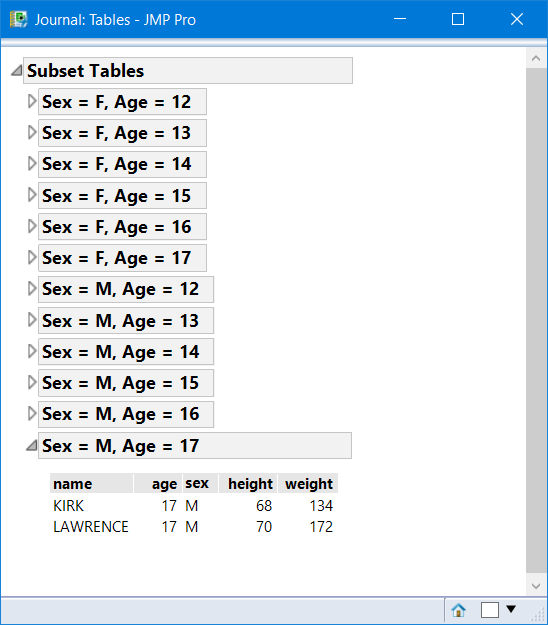Our World Statistics Day conversations have been a great reminder of how much statistics can inform our lives. Do you have an example of how statistics has made a difference in your life? Share your story with the Community!
Choose Language Hide Translation Bar
Highlighted

## Help With adding Data Tables in Journals using a loop.

Hi, I am new to JMP. I am tryiing to add tables generated by a loop into journal. But not having much luck. Any help with this would be appreciated. Here is my script. My initial table is being genarated by running SQL qurey.

dt2 = dt<<Summary(
Group( :MODULE, :STEP ),
Freq( "None" ),
Weight( "None" )
);

ModuleList = Column(dt2,"MODULE")<<Get Values;
StepList = Column(dt2,"STEP")<<Get Values;
numrows= nrows(dt2);
Close(dt2,nosave);

For (i=1, i<=numrows, i++,
dt<< select Where (:MODULE==ModuleList[i] & :STEP==StepList[i]);
odt= (dt << Subset(
Output Table( ModuleList[i] ),
Selected Rows( 1 ),
Selected columns only( 0 )));
dt<< clear select;
jrn<< Append(Text Box(ModuleList[i])); //Currently I am just adding the tables to journal.
odt<< journal; //However I would like to add them as Collesapble window/ListBox with ModuleList[i] as the title.
Close(odt,nosave);

);

2 ACCEPTED SOLUTIONS

Accepted Solutions
Highlighted

## Re: Help With adding Data Tables in Journals using a loop.

There are multiple methods to do this. Look up Help > Scripting Index > DataTableColBox for an alternative method.

Here is one method

``````Names Default to Here(1);

dt = Open("\$sample_data/Big Class.jmp");

//get the group list

Summarize(Data Table(dt), grps = By(:sex, :age) );

show(grps);  //List of 2 lists of equal size, the first list's items are gender, the second age

ob = OutlineBox("Subset Tables", vl=VListBox() );

For(i=1, i<=nitems(grps), i++,  //can also use grps
ttl = EvalInsert("Sex = ^grps[i]^, Age = ^grps[i]^");

//note Summarize lists converts numeric to character so num() function is required
//If the grouping columns are character, this is not needed.

dt << Select Where(:sex==grps[i] & :age==num(grps[i]) );
odt= (dt << Subset( Invisible, Output Table( ttl ),
Selected Rows( 1 ),
Selected columns only( 0 ))
);
dg = odt << New Data Box();
tmp = OutlineBox( ttl, dg);
tmp << close(1);
vl << append(tmp);
dt << clear select;
); // end for

//ob hase a nice table like view of each subset, but once you close the tables the view is gone
//so journaling creates te report view, and the subsetted tables can be closed.

nw = New Window("Tables", <<Journal);
ob << Journal;
Close All(Data Tables, Invisible, NoSave); ``````
Highlighted

## Re: Help With adding Data Tables in Journals using a loop.

Here is a screenshot of the Journal, with the last table opened.4 REPLIES 4
Highlighted

## Re: Help With adding Data Tables in Journals using a loop.

There are multiple methods to do this. Look up Help > Scripting Index > DataTableColBox for an alternative method.

Here is one method

``````Names Default to Here(1);

dt = Open("\$sample_data/Big Class.jmp");

//get the group list

Summarize(Data Table(dt), grps = By(:sex, :age) );

show(grps);  //List of 2 lists of equal size, the first list's items are gender, the second age

ob = OutlineBox("Subset Tables", vl=VListBox() );

For(i=1, i<=nitems(grps), i++,  //can also use grps
ttl = EvalInsert("Sex = ^grps[i]^, Age = ^grps[i]^");

//note Summarize lists converts numeric to character so num() function is required
//If the grouping columns are character, this is not needed.

dt << Select Where(:sex==grps[i] & :age==num(grps[i]) );
odt= (dt << Subset( Invisible, Output Table( ttl ),
Selected Rows( 1 ),
Selected columns only( 0 ))
);
dg = odt << New Data Box();
tmp = OutlineBox( ttl, dg);
tmp << close(1);
vl << append(tmp);
dt << clear select;
); // end for

//ob hase a nice table like view of each subset, but once you close the tables the view is gone
//so journaling creates te report view, and the subsetted tables can be closed.

nw = New Window("Tables", <<Journal);
ob << Journal;
Close All(Data Tables, Invisible, NoSave); ``````
Highlighted

## Re: Help With adding Data Tables in Journals using a loop.

Here is a screenshot of the Journal, with the last table opened.Highlighted

## Re: Help With adding Data Tables in Journals using a loop.

Hi @gzmorgan0 , Thank you for your reply. The solution was perfect. I just have one question. Is there a way to size the boxes(Outlinebox) so that the texts within the boxes stay in one line.
Thank you again for your time and help.

Highlighted

## Re: Help With adding Data Tables in Journals using a loop.

Instead of a VListBox, use a LineUpBox, that should help if the table titles are of different length.

If the tables are not subsets of teh same table, meaning the tables have varying number of columns, the only way I know how to do that is to use a container like a PanelBox for each of the tables. Find the larget width, then set each panel box the same width.

Hopefully, the LineupBox will be all that you need.  The changed script is attached.

Article Labels

There are no labels assigned to this post.